# Entropy Analysis of a Simple Rectangular Radiating Fin for Space Applications

Entropy Analysis of a Simple Rectangular Radiating Fin for Space Applications
Department of Mechanical Engineering, PES University, Electronic City Campus, Bangalore 560 100, India

Corresponding Author Email:
Page:
708-714
|
DOI:
https://doi.org/10.18280/ijht.380315
19 November 2019
|
Accepted:
10 June 2020
|
Published:
15 October 2020
| Citation

OPEN ACCESS

Abstract:

For growing interest in planetary exploration, it is substantial to study the utilization of energy for future-built planetary settlements, as energy resources are at a premium far away from earth. Entropy analysis is proposed as a suitable tool to study these systems, and make them energy efficient. Hence, a simple, purely radiating rectangular fin is studied by entropy generation analysis. The distribution of temperature and radiosity of the fin is first obtained by solving the governing heat transfer equation by second order finite difference numerical analysis. Using these data, the heat transfer and entropy generation of fin, fin-base, and the surrounding space is calculated. The effect of solar irradiation on the heat transfer of fin is also considered. The influence of pertinent, non-dimensional parameters on the total rate of entropy generated by the fin system is analyzed. Further, a correlation with these parameters is obtained for the total entropy generation.

Keywords:

1. Introduction

Every space system generates excess heat during operation which is to be rejected out, for the system to operate at its highest efficiency. One of the widely used, and an effective way, is to sculpt extended surfaces (fins) on the outer surface of the system’s physical build. The addition of fins increases the overall surface area which consequently increases the total rate of heat transfer. In space, we encounter only two ways of heat transfer i.e., conduction and radiation. The study of heat transfer from the system to the surrounding space via these fins is very essential for optimum functionality.

The effectiveness of a radiating fin was first studied by Bartas and Sellers . A review of sixty-five years of progress in extended surface technology has been published by Kraus . Heat transfer from convecting-radiating fins of different profile shapes was analysed by Nguyen . The scope of increasing the thermal efficiency of coated fins is examined by Plamondon . The optimization of radiative fin array system in a fuzzy environment is studied by Chung . An experimental analysis of radiating pin fins is performed by Murali .

The rate of heat transfer can be qualitatively analyzed by the second law efficiency. The ability of a system to do useful work is dependent on the available energy (exergy). Generally, the available energy is compromised by the conversion of useful energy into lower grade energy. This conversion is quantified by the term entropy. Thus, if the body generates entropy, available energy gets depleted. Adding fins to the surface of the system reduces the entropy generation rate of the body, thereby making it more efficient. As space modules have very limited energy resources, we have very little luxury for reduction in exergy. Therefore, it is critical to study the entropy analysis of the fin-base system for space applications.

The expression for increase in entropy of a system is given by:

$d s=\left(\frac{\delta Q}{T}\right)_{\text {reversible }}>0$       (1)

An ideally functioning fin has constant temperature throughout the length of extension, which is equal to its base temperature . The second law analysis of different heat transfer models is given by Bejan . Extensive work has been done for fins which rejects heat by forced convection , and convection and radiation , for earth atmospheric conditions. However, research done on purely radiating fins is sparse.

Radiating fins have a large variety of space applications such as the thermal control system in lunar modules, satellite radiators, space probes, etc., and it is crucial to study the working and optimization of these fins for future deep space missions, and exploration of other planets. Application and analysis of various fin types as radiation heat sink on printed circuit boards is given by Basyigit et. al. . Mass is at a premium when it comes to orbital analysis. However, for planetary and lunar stations, energy conservation plays a vital role. Mass optimization of thin rectangular fins is researched by Wilkins . Also, optimization of the design of space radiating elements in terms of heat rejected per unit mass has been developed by Naumann . Here, we focus our research on energy preservation. Entropy analysis on plain tubular space radiators was done by Balaji and Venkateshan . Adding fins on these radiator tubes increases the overall efficiency.

In this paper, we perform a detailed study on a single rectangular, radiating extended surface with an adiabatic tip. The conduction-radiation interaction heat exchange problem is formulated using radiosity-irradiation method after calculation of relevant view-factors between the base and the fin surface. The transfer of heat from the heated base to the surrounding space via the fin results in increasing the entropy of the universe. The heat transfer mechanism and the rate of entropy generated by the system is mathematically formulated, and solved by finite difference numerical approximation. The effect of various parameters on the rate of entropy generation is extracted, and the supporting correlation is developed. The influence of fin and environmental parameters on the rate of entropy generation is analyzed, and conditions for low entropy generation can be deduced. The entropy analysis aims to provide a basis for optimized heat rejection with minimum irreversibility, thus maximizing overall conservation of useful energy in the system. The study is applicable for space heat rejection systems where energy resources are at a premium, like a lunar base.

2. Formulation and Analysis

A simple rectangular fin of length L and thickness t (t<<L), and width w, placed on a flat surface is considered for this study, as shown in Figure 1. As the thickness is very less compared to the length of fin, we consider the conduction to occur in one dimension only - along the length of the fin. To consider radiation exchange with the base surface, the length of base surface is taken as ten times the fin’s length, for a conservative approach. The base of the fin is assumed to be maintained at constant temperature Tb. The fin is considered to lose heat only by radiation to the space at 4 K, and also has significant mutual radiation exchange with the base surface. The radiation interaction is modelled using radiosity-irradiation method. The fin is also considered to be exposed to solar irradiation at a given angle.

## 1.jpgFigure 1. Fin schematic

## 2.png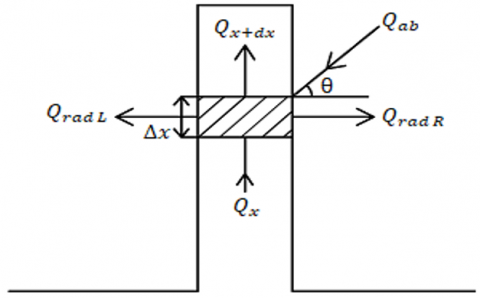Figure 2. 1-D heat flow diagram

With reference to Figure 2, the governing equation under steady state is derived for a differential element shown, by applying conservation of energy principle (Eq. (2) - Eq. (7b)),

$Q_{x}-Q_{x+d x}-\left(Q_{\text {radL} }+Q_{\text {radR}}\right)+Q_{a b}=0$        (2)

Using Fourier’s law of conduction, subsequently applying Taylor series expansion and retaining only first order terms , we get

$Q_{x}-Q_{x+d x}=k w t \frac{d^{2} T}{d x^{2}} d x$      (3)

Heat transfer leaving a surface by radiation is given by,

$Q_{r a d}=\frac{w \Delta x \varepsilon}{(1-\varepsilon)}\left(\sigma T^{4}-J\right)$       (4)

Heat absorbed from the solar irradiation is given as,

$Q_{a b}=\alpha I A_{\text {projected}}$      (5)

where, the projected area of the differential element, $A_{\text {projected}}=w \Delta x \cos \theta$, is the area available for the absorption of solar irradiation which is impinging at an angle θ, normal to the fin surface.

By substituting Eqns. (3-5) in the energy balance equation Eq. (2), and simplifying we get,

$\frac{d^{2} T}{d x^{2}}-\frac{\varepsilon}{(1-\varepsilon) k t}\left(2 \sigma T^{4}-J_{L}-J_{R}\right)+\frac{\alpha I \cos \theta}{k t}=0$      (6)

For the ease of analysis and reduction in the number of parameters for the parametric study, Eq. (6) is non-dimensionalized by defining the following non-dimensional parameters,

$\theta=\frac{T}{T_{b}}, z=\frac{\Delta x}{L}, N_{r}=\frac{\sigma L^{2} T_{b}^{3}}{k t}, \jmath=\frac{J}{\sigma T_{b}^{4}}, \theta_{r e f}^{4}=\frac{\alpha I \cos \theta}{\sigma T_{b}^{4}}$        (7a)

Considering symmetry (JL=JR), (Eq. (6)) in a non-dimensional form becomes

$\frac{d^{2} \theta}{d z^{2}}-\frac{\varepsilon N_{r}}{(1-\varepsilon)}\left(2 \theta^{4}-2 \jmath\right)+N_{r} \theta_{r e f}^{4}=0$       (7b)

The governing differential equation, Eq. (7b), when solved gives the non-dimensional temperature distribution in the fin, however only after non-dimensional radiosities (ℐs’) are calculated for each differential element. The differential equation, Eq. (7b) is approximated by a second order finite difference method for solution. The fin is divided into N+1 number of nodes along the length of the fin, and the above mentioned radiosities are calculated using radiosity-irradiation method as follows :

The non-dimensional radiosities of N+1 number of differential elements on the fin, and the base surface, which is the (N+2)nd surface, are given by,

$j_{i}=\varepsilon \theta_{i}^{4}+(1-\varepsilon) \sum_{j=1}^{N+3} F_{i j} \jmath_{j} ; 1 \leq i \leq(N+2)$     (8a)

where, space is the (N+3)rd surface, and Fij is the view-factor between the ith and jth surfaces. The space is considered to be a black body with ε=1, hence radiosity of space becomes,

$\mathcal{J}_{N+3}=\theta_{\text {space}}^{4}$      (8b)

where, $\theta_{\text {space}}=\frac{T_{\text {space}}}{T_{b}}$.

The calculation of radiosities requires (N+3)x(N+3) view factor matrix. These view-factors are calculated using configuration C-14 , and view-factor algebra. Once all the relevant view-factors are calculated, (N+2) number of radiosities are estimated using Gauss-Seidel iterations by solving (N+2) number of simultaneous algebraic equations given by Eq. (8a) when temperatures are specified.

In order to obtain temperature distribution of the fin, a global iterative procedure is used to solve Eq. (7b). Initially, values are assumed for all nodal temperatures. With these assumed temperatures, Eq. (8a) is solved using Gauss-Seidel iterations and radiosities are obtained. Using these radiosities, Eq. (7b) is solved using second order finite difference method and new values of nodal temperatures are obtained. These new values are compared with the initially assumed values and global iterations are continued until convergence.

Having obtained the non-dimensional temperature distribution, the non-dimensional heat transfers and entropy generation of the system are calculated.

Non-dimensional heat transfer rates of the fin and base surfaces are obtained as,

$\phi_{\text {base}}=\frac{Q_{\text {base}}}{A_{s} \sigma T_{b}^{4}}=\frac{\varepsilon A_{\text {base}}}{(1-\varepsilon) A_{s}}\left(1-\mathcal{J}_{\text {base}}\right)$    (9)

$\phi_{f i n}=\frac{Q_{f i n}}{A_{s} \sigma T_{b}^{4}}=\sum_{i=1}^{N+1} \frac{\varepsilon}{(1-\varepsilon)}\left(\theta_{i}^{4}-\mathcal{J}_{i}\right)$      (10)

According to the second law, the entropy generation rates of the fin, base surface and surrounding space are calculated individually, and algebraically added to obtain total entropy generated by the fin-base system for analysis (Eq. (13)-Eq. (17)).

Entropy generated by the surrounding space is given by,

$_{\text {space }}=\frac{Q_{\text {base}}+Q_{\text {fin}}}{T_{\text {space}}}$      (11)

Entropy generated by the base surface of the fin is given by,

$_{\text {base}}=\frac{Q_{\text {base}}}{T_{b}}$      (12)

Non-dimensionalizing, we get,

$S_{\text {space }}=\frac{\text{Ȿ}_{ space }}{A_{s} \sigma T_{b}^{3}}=\frac{\phi_{\text {fin}}+\phi_{\text {base}}}{\theta_{\text {space}}}$         (13)

$S_{\text {base}}=\frac{-\text{Ȿ}_{\text {base}}}{A_{s} \sigma T_{b}^{3}}=-\phi_{\text {base}}$       (14)

Since the temperature is not constant along the fin, entropy generation of each differential element is calculated and summated to obtain the cumulative entropy generated by the entire fin.

Ȿ$_{f i n}=\sum_{i=1}^{N+1} \frac{\frac{-w \Delta x \varepsilon}{(1-\varepsilon)}\left(\sigma T_{i}^{4}-J_{i}\right)}{T_{i}}$      (15)

Non-dimensionalizing,

$S_{f i n}=\frac{\text{Ȿ}_{f i n}}{A_{s} \sigma T_{b}^{3}}=\frac{-\varepsilon}{(1-\varepsilon)} \sum_{i=1}^{N+1}\left(\frac{\theta_{i}^{4}-\mathcal{J}_{i}}{\theta_{i}}\right)$       (16)

Total non-dimensional rate of entropy generated by the system is given by,

$S_{\text {gen}}=\left(S_{\text {space}}+S_{\text {base}}+S_{\text {fin}}\right)$       (17)

which is always positive following second law of thermodynamics. Hence, the non-dimensional total entropy generation by the system can be any non-zero positive number.

3. Results and Discussion

From the second law of thermodynamics, the entropy generation increases with the increase in heat transfer, however the second law efficiency dictates that an irreversible system can be more efficient if the irreversibility is minimized. Based on this theory, the effect of certain characteristic fin parameters on rate of entropy generation is observed by numerical analysis. The fin-base temperature of 393.15K, and the outer space temperature of 4K is considered for this study. The range of parameters considered in the present study is given in Table 1.

Table 1. Range of parameters considered for study

 Parameters Range Non-dimensional Radiation-conduction interaction parameter (Nr) 10-3–102 Emissivity (ε) 0.2 – 0.8 Non-dimensional Environmental parameter ($\theta_{r e f}^{4}$) 0 – 0.21 Base length Lb 10-4–100(m) Base temperature Tb 393.15 (K) Space temperature Tspace 4 (K) Fin thickness (t) 0.002 (m)

## 3.png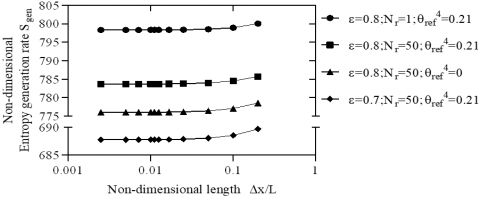Figure 3. Grid study for finite difference analysis

Before we proceed to the parameter study, a grid study concluded that the variation of the rate of total entropy generated with the non-dimensional length of the fin is minimal up to10-2, for different values of radiation-conduction interaction parameter (Nr), emissivity (ε), and environmental parameter $\left(\theta_{r e f}^{4}\right)$ as shown in Figure 3. Thus, the non-dimensional length is fixed to 10-2 for all calculations.

The numerical analysis is validated by comparing results with a standard textbook with set parameters , having Nr=0.64, L=0.15 m, Tb=373 K, ε=0.8 and $\theta_{r e f}^{4}$=0.575011; non-dimensional temperature v/s non-dimensional length graph was plotted and compared, resulting an average error of 0.306172%, as shown in Figure 4.

## 4.png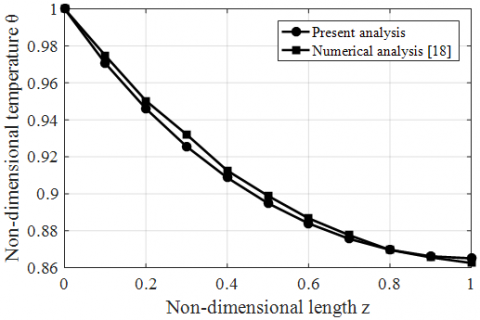Figure 4. Validation of numerical analysis

3.1 Effect of radiation-conduction interaction parameter

The non-dimensional radiation-conduction parameter (Nr), helps us give the relative state at which heat is being transferred in the fin-base system. If Nr is greater than unity, it implies that heat transfer by radiation is more dominant than heat transfer by conduction in the system. Conversely, if Nr is less than 1, rate of conduction is more dominant. The effect Nr on the rate of entropy generated is studied by fixing the physical dimensions of the fin, i.e., w=1 m, and the base surface Lb=Rb=10m. Figure 5 shows the changes in total rate of entropy generated by the system for the variation of radiation-conduction interaction parameter from 10-3 to 10-2, for two different values of emissivity, with and without solar irradiation. Total entropy generated by fin-base system (Sgen), remains almost constant from 10-3 to 10-1 of Nr due to predominant heat transfer by conduction in the fin, relative to heat lost by radiation. Hence, there is a build-up of heat in the fin system. Further, there is a non-linear decrease in the rate of entropy generation from 10-1 to 1 of Nr, due to the gradual increase in heat transfer by radiation via the fin. Sgen becomes almost constant for greater values of Nr due to the inability of heat transfer from base surface to the fin. Hence, majority of the radiation occurs from the base surface. From Figure 5, the average maximum change in Sgen  throughout the range of Nr is 5.54%. Therefore, we can conclude that the effect of radiation-conduction parameter for the observed range is moderate. On further analysis, the graph characteristics remains same for different values of emissivity and environmental parameter $\left(\theta_{r e f}^{4}\right)$. Material having higher emissivity has a higher rate of entropy generation, due to increased rate of heat loss to the surrounding space. There is almost no influence of the environmental parameter on total entropy generated by the fin-base system from 10-3 to 10-1 of Nr due to extensive heat build-up. From 10-1 of Nr, variation of Sgen can be observed for different values of $\theta_{r e f}^{4}$ which is caused by increase in radiation heat transfer. For non-zero $\theta_{r e f}^{4}$ values, the fin’s ability to lose heat is decreased due to absorption of background radiation, consequently increasing the entropy generation rate. We can also deduce that the variation of emissivity has a greater impact on Sgen than the varying environmental parameter. From Figure 5, a variation of only 1.176% is observed for Sgen when $\theta_{r e f}^{4}$ is varied between 0 and 0.21 (at Nr=100, keeping emissivity constant). Whereas, a variation of 14.153% is observed by varying emissivity from 0.7 to 0.8 (at Nr=100, keeping $\theta_{r e f}^{4}$ constant).

## 5.png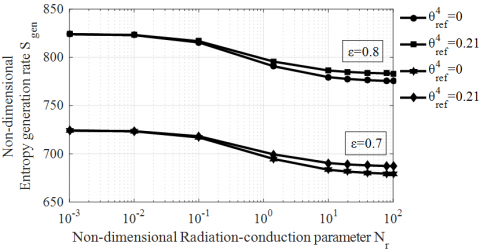Figure 5. Effect of radiation-conduction interaction parameter (Nr), on non-dimensional entropy generated by the fin base system

3.2 Effect of emissivity

The effect of emissivity on the rate of entropy generated is studied for a fin of unit width. The base length on either side is set to 10 m, to account for the significant radiation exchange between the fin and base. The emissivity (ε), is varied from 0.2-0.8, and the result is observed for different values of radiation-conduction interaction parameter (Nr) and environmental parameter $\left(\theta_{r e f}^{4}\right)$. From Figure 6, the total rate of entropy generation increases almost linearly with the increase in emissivity of the fin material, as more heat is lost to the space due to increased emission from fin material. Intuitively one might assume, increased heat transfer is key to increasing efficiency of the fin-base system. However, this assumption is disproved by the second-law efficiency. Therefore, in order to increase efficiency, the system should be in a low entropy generating state. From Figure 6, the average change in Sgen is 19.76%, for every 0.1 change in ε (all cases considered). Thus, we can conclude that the influence of ε for the observed range is drastic. Furthermore, from graph we can deduce that Nr and $\theta_{r e f}^{4}$ parameters have little effect on the overall rate of entropy generation, when compared to varying emissivity.

## 6.png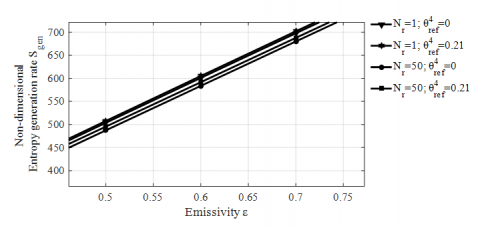Figure 6. Effect of emissivity on non-dimensional rate of entropy generated by the fin base system

On the other hand, entropy generated by fin has a non-linear increase in emission of heat, due to the adverse effect of fin-base interaction, as shown in Figure 7. Entropy generation rate of fin for low Nr values is greater as conduction supersedes radiation. The increased conduction traps the surface heat, consequently making the fin have a higher temperature distribution, which in turn reduces its efficacy. The presence of solar irradiation further compounds this situation (for non-zero $\theta_{r e f}^{4}$ values). On further observation, Sfin for Nr=50 is almost constant implying that, increasing emissivity has little to no effect on entropy generation rate. Since heat transfer by radiation is high for Nr=50, the fin is at a low temperature, thereby having low values of Sfin. In this case, the Sfin is highly influenced by the environmental parameter, which is shown by keeping Nr  constant at 50, and varying $\theta_{r e f}^{4}$.

## 7.png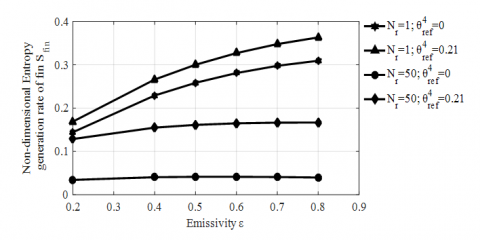Figure 7. Effect of emissivity on non-dimensional rate of entropy generated by the fin

3.3 Effect of base length

The effect of fin base length on the entropy generated by the system is studied. The width of fin and fin base is fixed to 1m. Also, the emissivity of the fin material is taken as 0.8. Figure 8 shows the variation of total entropy generation rate of fin-base system with increasing base length, for two different values of Nr, with and without solar irradiation. It is observed that the rate of entropy generation remains almost constant with respect to increasing base length till around 0.1 m. After that, there is a significant increase in entropy generation because heat lost by the base becomes more significant as its surface area is increased. Entropy generation rate is higher for low Nr value. This is caused by the relatively high conduction rate, which inhibits the rejection of heat to the surrounding space. From Figure 8, a decrease of 58.754% of Sgen is observed when Nr is varied from 1 to 50 (for constant $\theta_{r e f}^{4}$=0.21), at base length equal to 10-4. For non-zero value of environmental parameter $\left(\theta_{r e f}^{4}\right)$, Sgen is higher due to increased absorption of radiation from the surrounding space, which further reduces the capability of fin to expel heat. The effect of environmental parameter for Nr=1 on entropy generation rate is lesser than for Nr=50. This variation is caused due to difference in rate of heat rejection i.e., for higher Nr value, the rate of heat rejection by radiation is more and the environmental parameter acting in this scenario reduces the radiation rate, thereby making the variation of environmental parameter with respect to Sgen more prominent. By contrast, for increased conduction rates the environmental parameter has less effect on the entropy generation rate. At base length equal to 10-4, Sgen increases by 65.27% for Nr=50, but an increase of just 18.33% is observed for Nr=1. Therefore, environmental parameter plays a greater role when the fin is under high radiating state.

Also, a gradual decreases in entropy generation rate of fin (Sfin) can be observed in Figure 9 by varying base length from 0.01 m to 1 m. The average decrease of 32.5% of Sfin is observed, which is caused by the ineffectiveness of the fin as most of the heat is lost by the base surface making the fin redundant with increase in base-length.

## 8.png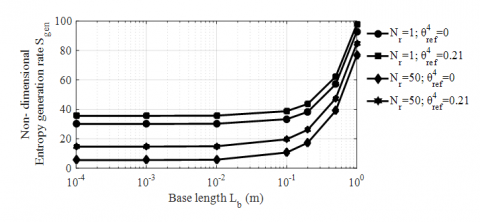Figure 8. Effect of base area on non-dimensional total rate of entropy generated

## 9.png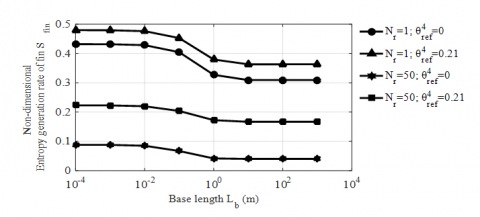Figure 9. Effect of base area on non-dimensional rate of entropy generated by the fin

4. Correlation

In order to present the study in a much usable form, a non-linear regression for the relevant parameters is carried out to generate a correlation for non-dimensional total entropy generation rate of the fin-base system (Sgen). The range of parameters considered for the regression analysis is given in Table 2. The correlation developed, based on 500 data points obtained based on the parametric study is given by,

$S_{g e n}=969.50725814\left(\varepsilon^{0.95166843}\right)\left(N_{r}^{-0.00386201}\right)\left(1+\theta_{r e f}^{4}\right)^{0.07466929}$      (18)

The correlation coefficient is found to be 0.99932, with an RMS error of 6.38%. The parity plot shows no bias as shown in Figure 10.

## 10.pngFigure 10. Parity plot showing no bias between numerical and correlated Sgen

By observing the power magnitude of each parameter in the above correlation equation, we can clearly state that the total entropy generation rate is strongly dependent on emissivity. The effect of Nr is considerably less as heat transfer by base surface is dominant. Furthermore, the environmental parameter ($\theta_{\text {ref}}^{4}$) shows moderate influence.

Table 2. Range of parameters considered for correlation

 Parameters Range Nr 0.1 – 100 ε 0.1 – 0.9 $\theta_{\text {ref}}^{4}$ 0 – 0.96
5. Conclusions

A rectangular fin exposed to non-atmospheric conditions for space application, is analysed using minimum exergy (available energy) loss concept and the following conclusions are drawn:

1) The radiation-conduction interaction parameter Nr, showed moderate influence on Sgen. For the range considered in the study, high Nr values resulted in a decrease of 5.54% in total rate of entropy generation. The environmental parameter started to show its effect only for Nr>0.1.

2) The influence of emissivity on total rate of entropy generation is more prominent than other parameters considered. An almost linear relation is observed for the increase in Sgen with respect to increase in emissivity. An average increase of 19.76% of Sgen is observed for every 0.1 increase in emissivity. Therefore, lowering the emissivity of the fin material will significantly lower the Sgen, thereby increasing the overall efficiency of the system, however at the cost of reduced heat transfer.

3) The influence of environmental parameter $\left(\theta_{r e f}^{4}\right)$ showed significant rise in Sgen, but relatively less, when compared to the effect of emissivity. This can be observed in the power associated with $\theta_{r e f}^{4}$ in the developed correlation. Furthermore, the influence of $\theta_{r e f}^{4}$ increases when the fin is under high radiating state, i.e., for Nr>0.1.

4) Sgen rose exponentially for Lb>0.1 m, therefore it is advised to limit the surface area surrounding the fin for increased exergy.

5) The correlation with the relevant parameters is presented for easy calculation of dimensionless entropy generation rate of the fin-base system (Sgen), as a function of non-dimensional fin parameters.

Nomenclature
 Lb Base length on the left side of fin, m Rb Base length on the right side of fin, m t Thickness of fin, m L Length of fin, m N Number of divisions made to discretize fin w Width of fin, m Ac Cross-sectional area of fin, m2 As Radiating surface area of fin, m2 Q Heat transfer rate, W k Thermal conductivity, W.m-1. K-1 Tb Base temperature, K z Non-dimensional length Nr Radiation-conduction interaction parameter F View-factor J Radiosity, W.m-2 Ȿ Rate of entropy generated, W.K-1 S Dimensionless rate of entropy generation I Solar irradiation, W.m-2 Greek symbols σ Stefan-Boltzmann constant, W.m-2. K-4 α Solar absorptivity Δx Discretized element length of fin, m ε Emissivity $\phi$ Dimensionless heat transfer rate θ Dimensionless temperature $\theta_{\text {ref}}^{4}$ Environmental parameter $\jmath$ Dimensionless radiosity Subscripts L Corresponding to left surface of fin R Corresponding to right surface of fin base Corresponds to base surface fin Corresponds to fin space Corresponds to surrounding space gen Corresponds to total quantity
References

 Bartas, J.G., Sellers, W.H. (1960). Radiation fin effectiveness. Journal of Heat Transfer, 82(1): 73-75. https://doi.org/10.1115/1.3679882

 Kraus, A.D. (1962). Sixty-Five years of extended surface technology (1922-1987). Applied Mechanics Reviews, 41(9): 321-364. https://doi.org/10.1115/1.3151910

 Nguyen, H., Aziz, A. (1992). Heat transfer from convecting-radiating fins of different profile shapes. Wärme-Und Stoffübertragung, 27(2): 67-72. https://doi.org/10.1007/bf01590120

 Plamondon, J.A. (1962). Thermal efficiency of coated fins. Journal of Heat Transfer, 84(4): 279-282. https://doi.org/10.1115/1.3684371

 Chung, B.T.F., Zhang, B.X., Lee, E.T. (1996). A multi-objective optimization of radiative fin array systems in a fuzzy environment. Journal of Heat Transfer, 118(3): 642-649. https://doi.org/10.1115/1.2822680

 Ganesh Murali, J., Katte, S.S. (2007). Experimental study of threaded and grooved radiating pin fin. ASME/JSME 2007 Thermal Engineering Heat Transfer Summer Conference, 3: 763-769. https://doi.org/10.1115/HT2007-32043

 Hesselgreaves, J.E., Law, R., Reay, D. (2017). Chapter 7 - thermal design. Compact Heat Exchangers (Second Edition), 275-360. https://doi.org/10.1016/b978-0-08-100305-3.00007-0

 Bejan, A. (1980). Second law analysis in heat transfer. Energy, 5(8-9): 720-732. https://doi.org/10.1016/0360-5442(80)90091-2

 Balaji, C., Venkateshan, S.P. (1996). Thermodynamic optimization of tubular space radiators. Journal of Thermophysics and Heat Transfer, 10(4): 705-707. https://doi.org/10.2514/3.850

 Poulikakos, D., Bejan, A. (1982). Fin geometry for minimum entropy generation in forced convection. Journal of Heat Transfer, 104(4): 616-623. https://doi.org/10.1115/1.3245176

 Basyigit, I.B., Genc, A., Dogan, H., Helhel, S. (2020). The effect of fin types of the heatsinks on radiated emission on the printed circuit board at S‐C band. Microwave and Optical Technology Letters, 62(10): 3099-3106. https://doi.org/10.1002/mop.32420

 Wilkins Jr, J.E. (1960). Minimizing the mass of thin radiating fins. Journal of the Aerospace Sciences, 27(2): 145-146. https://doi.org/10.2514/8.8436

 Naumann, R.J. (2004). Optimizing the design of space radiators. International Journal of Thermophysics, 25(6): 1929-1941. https://doi.org/10.1007/s10765-004-7747-0

 Sasikumar, M., Balaji, C. (2002). A holistic optimization of convecting-radiating fin systems. Heat Transfer, 124(6): 1110-1116. https://doi.org/10.1115/1.1497358

 Han, J.C. (2016). Analytical Heat Transfer, Heat Conduction Equations. CRC Press.

 Sparrow, E.M., Cess, R.D. (1966). Radiation Heat Transfer, Wadsworth Publishing Company, Belmont, Calif.

 Howell, J.R. (2010). A Catalog of Radiation Heat Transfer Configuration Factors. 3rd Edition.

 Venkateshan, S.P. (2011). Heat Transfer. 2nd Edition, Ane Books Pvt. Ltd. Publications.